## Monday, November 10, 2014

### Data Mining in Excel Part 28: Classification Matrix

Today, we're continuing our discussion about Model Validation by looking at the Classification Matrix.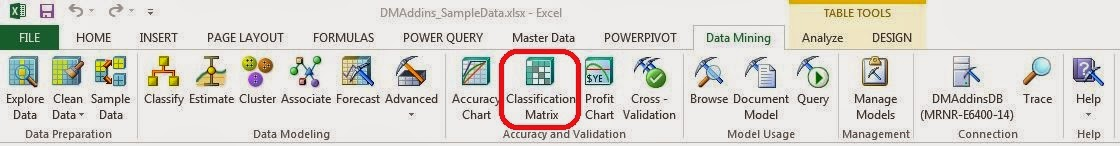Classification Matrix
A Classification Matrix is very useful for determine how well a model predicts values by comparing the predictions to the known values.  Simply put, there are four outcomes for a binary (Yes/No) variable.  First, the actual value is No and the prediction is No (this would be a correct prediction).  Second, the actual value is Yes and the prediction is Yes (this would also be a correct prediction).  Third, the actual value is No and the prediction is Yes (this would be an incorrect prediction and a Type I Error).  Lastly, the actual value is Yes and the prediction is No (this would be an incorrect prediction and a Type II Error).  For more information on Type I and Type II Errors, read this.  A good model reduces the chance of error and increases the chance of correct predictions.  We can display this in a simple grid format.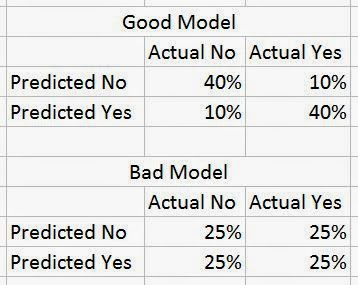Classification Matrices (Mock-up)
The first model has a high chance (80%) of correct predictions (No/No and Yes/Yes) and a low chance (20%) of incorrect predictions (No/Yes and Yes/No), while the bad model only has a 50/50 chance of predicting the correct value.  There's not really a golden rule for how well a model needs to be able to predict values.  For instance, it's not fair to say that a model needs at least a 75% chance of predicting correctly in order to called "Good".  How much is good enough depends on the data and the problem.  For instance, in some marketing campaigns, you have success rates at low as 5% or 10%.  The only thing needed for a good model is that it gleams reasonable insight from actual data.  Everything else comes from good data stewardship and model validation.  Let's see it in action.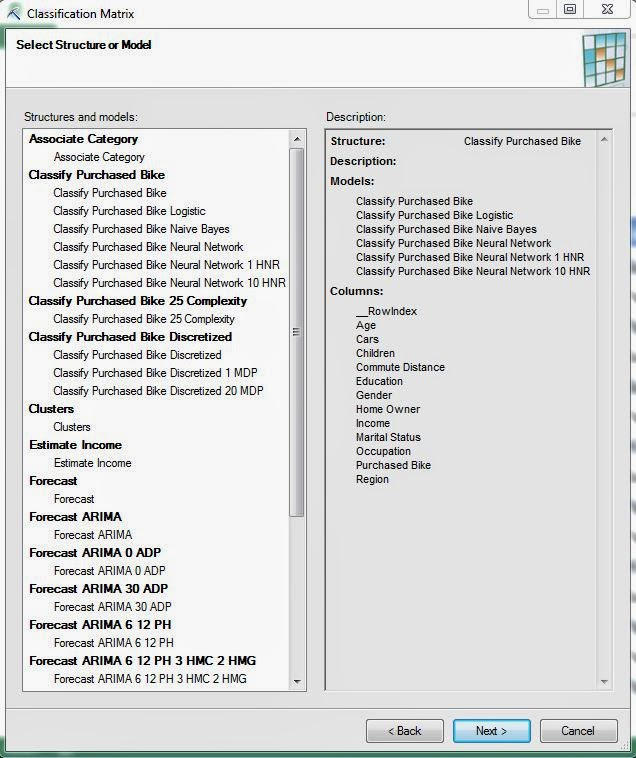Select Structure
The first thing we need to do is pick a model or structure that we would like to create some classification matrices for.  A classification matrix is perfectly valid for a single model, but they are definitely better if you can compare multiple models.  Just like the previous post on Accuracy Charts, this technique will not work on models built using the Association Rules or Time Series algorithms.  Let's keep going.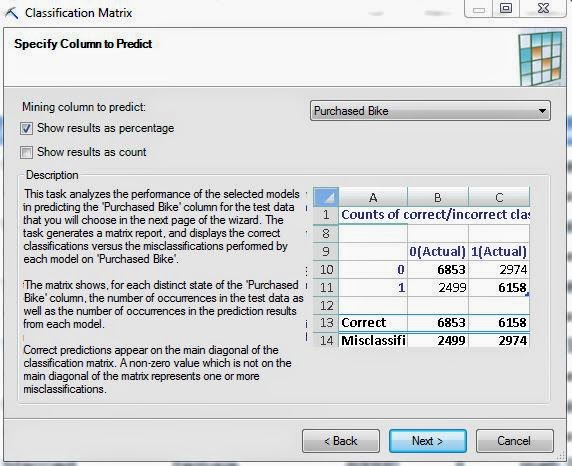Select Column
The next step is to select which column we would like to predict.  Since this structure only has one predictable column, we don't have much choice.  However, we do have the option of seeing percentages, counts, or both.  Personally, we like to see percentages, but the choice is yours.  Let's move on.Select Data Source
Again, we have the option of choosing a data source.  We defined test data for exactly this reason; so, there's no harm in using it.  Just remember, it's not acceptable to use the same data that you used to train the model.  Let's check out the results.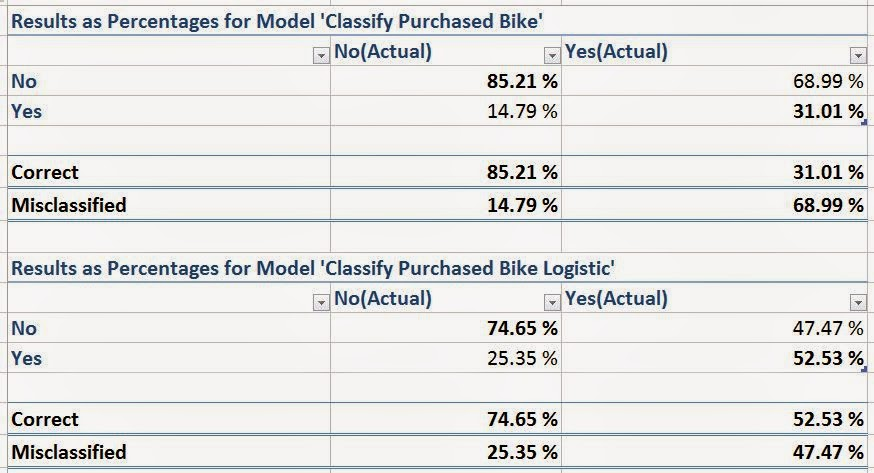Classification Matrices (Purchased Bike)
We have a classification matrix for each model in the structure.  The percentages in these matrices are calculated slightly differently than in our mock-up.  In these matrices, each column adds up to 100%.  This is because we can conceptualize each Actual value as a unique outcome.  After all, a single person can't be a bike buyer while also not being a bike buyer.  We prefer this method, but there are quite a few others as well.  You're free to google them if you want.  Just on the first page of results we saw at least four distinct types of classification matrices.

An important thing to note from these models is how well they predict the outcome you are looking for.  In our case, we want to find bike buyers.  We don't care about the non-bike buyers.  So, all we need to focus on is the Yes column.  In that case, the Logistic Model (the bottom one) seems to be a pretty poor model.  It's barely better than flipping a coin!  However, if you compare it to the Decision Tree Model (the top one), you'll see that 52% is not a bad percentage.  Let's take a look at the last type of chart.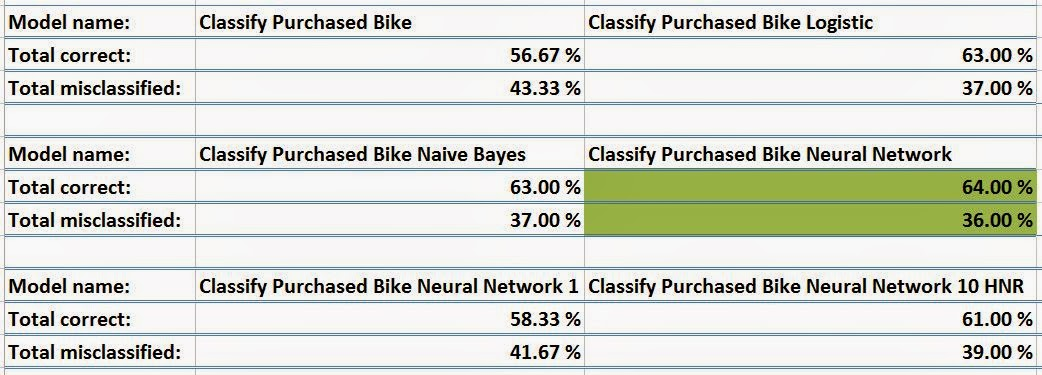Multi-Model Classification Matrix
This matrix was originally on a single line in Excel.  However, it was difficult to screenshot.  So, we used some Excel magic to put it on multiple lines.  This matrix shows us how well each model compares, regardless of which outcome we care about.  It also highlights the "best" model in green.  If we look back down at our single model classification matrices, we see that the Naive Bayes Model actually has the highest Yes/Yes classification percentage at 53.8%.

So, the accuracy chart told us to use the Logistic Model, the single-model classification matrix told us to use the Naive Bayes Model and the multi-model classification matrix told us to use the Neural Network Model.  Which model is the right one?  There's no real answer to that question.  It's up to each of us to make that decision on our own.  In the real world, there is rarely a "best" answer.  Simply choose the model that works well for you.  Keep an eye out for our next post where we'll be talking about Profit Charts.  Thanks for reading.  We hope you found this informative.